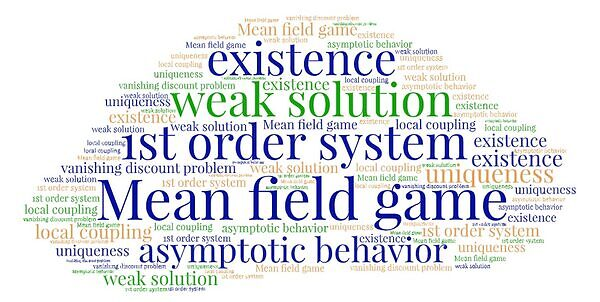# On weak solutions to first-order discount mean field games### Date

Friday, October 8, 2021 - 10:00 to 11:00

Zoom seminar

### Description

Title: On weak solutions to first-order discount mean field games

Speaker: Hiroyoshi Mitake, University of Tokyo

Abstract: In this talk, we consider a stationary first-order mean field game system with a local coupling, and first establish the existence and uniqueness of weak solutions. Our main interest of this paper is to study the asymptotic behavior of the solution $(u^\varepsilon,m^\varepsilon)$ as the discount  factor $\varepsilon \to 0$. We call this asymptotic problem the vanishing discount problem. The vanishing discount problem for Hamilton-Jacobi equations has been recently developed well. Analogically, we can naturally expect that the limit problem is described by the associated  ergodic problem. One of main features of this asymptotic problem is that the ergodic problem may have multiple weak solutions. Indeed, we show an example to illustrate the multiplicity of weak solutions to the ergodic problem. This fact makes the asymptotic problem delicate and interesting. We show a necessary condition that any limit of solutions under subsequence satisfies. By using this condition, we show a nontrivial example to get the convergence result. This is a joint work with K. Terai (Univ. of Tokyo).